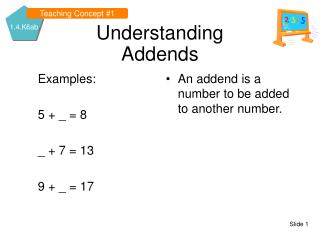DownloadDownload PresentationDownload Presentation- - - - - - - - - - - - - - - - - - - - - - - - - - - E N D - - - - - - - - - - - - - - - - - - - - - - - - - - -
##### Presentation Transcript

1. Examples: 5 + _ = 8 _ + 7 = 13 9 + _ = 17 An addend is a number to be added to another number. Teaching Concept #1 UnderstandingAddends

2. Examples: 5 + 3 = _ 6 + 7 = _ 9 + 8 = _ Sum – the value that results from the addition of two or more numbers. Identify the addends and the sums: 4 + 5 = 9 12 = 3 + 9 Teaching Concept #2 UnderstandingSums

3. Examples: 8 - _ =3 13 - _ = 7 17 - _ = 8 A subtrahend is a number subtracted from another number. Teaching Concept #3 Understanding Subtrahends

4. Examples: 8 – 5 = _ 13 – 6 = _ 17 – 9 = _ Difference – the value that results from the subtraction of one number from another. Identify the subtrahends and differences: 10 – 7 = 3 9 – 2 = 7 Teaching Concept #4 UnderstandingDifferences

5. Examples: 5 x _ = 15 7 x _ = 42 9 x _ = 72 A factor is a number that is multiplied by another number. Teaching Concept #5 UnderstandingFactors

6. Product – the value that results from the multiplication of two or more numbers. Identify the factors and the products: 2 x 6 = 12 3 x 3 = 9 Examples: 5 x 3 = _ 7 x 6 = _ 9 x 8 = _ Teaching Concept #6 Understanding Products

7. What is the inverse of : Addition? Subtraction? A fact family is a group of related facts using the same three numbers. Let’s check our work by using fact families. 5 + 3 = 8 3 + 5 = 8 {8 – 5 = 3 8 – 3 = 5} 13 – 6 = 7 13 – 7 = 6 {6 + 7 = 13 7 + 6 = 13} Teaching Concept #7 UnderstandingFact Families

8. Using repeated addition, solve the multiplication equation. 5 + 5 + 5 = __ 3 x 5 = __ Now check your answer: 5 + 5 + 5 = 15 3 x 5 = 15 Using repeated addition with multiplication

9. Megan and Mallory have kittens. Megan has five kittens and Mallory has three kittens. How many kittens do the two girls have altogether? 5 + 3 = _ Application of Concept

10. Application of Concept • Megan and Mallory have eight kittens between them. Megan has five kittens. How many kittens does Mallory have? • 8 – 5 = _

11. Michael had three hits during each of his first five baseball games. How many hits did Michael have in all? 3 + 3 + 3 + 3 + 3= _ 5 x 3 = __ Application of Concept

12. Concrete Example #1 Modeling of New Concept

13. Step-by-Step #1 Demonstration 5 + 3 = 8

14. Concrete Example #2 Modeling of New Concept

15. Step-by-Step #2 Demonstration 3 + 3 + 3 + 3 + 3 = 15